Ex 6.2

Chapter 6 Class 12 Application of Derivatives
Serial order wise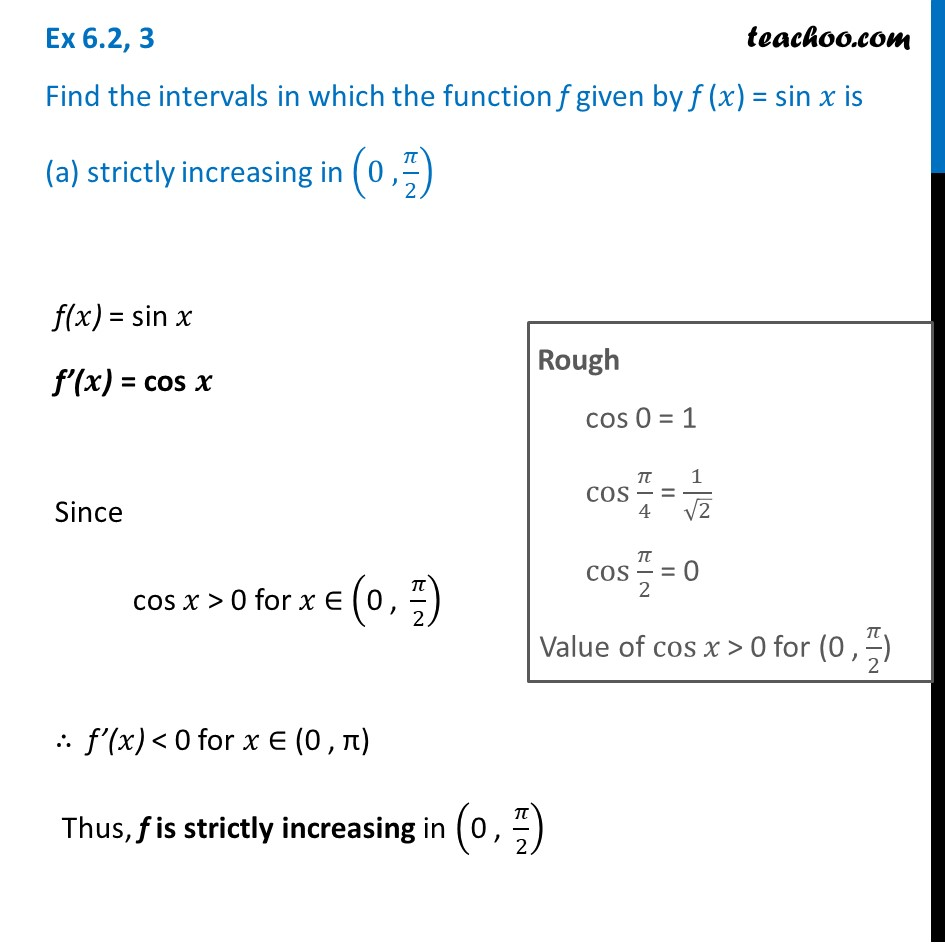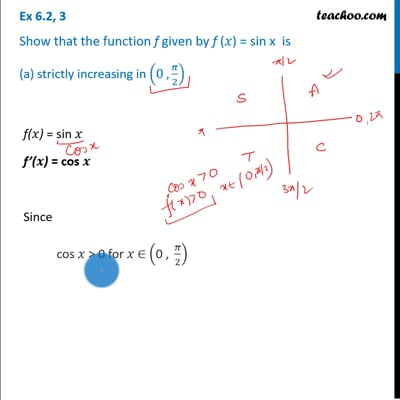This video is only available for Teachoo black users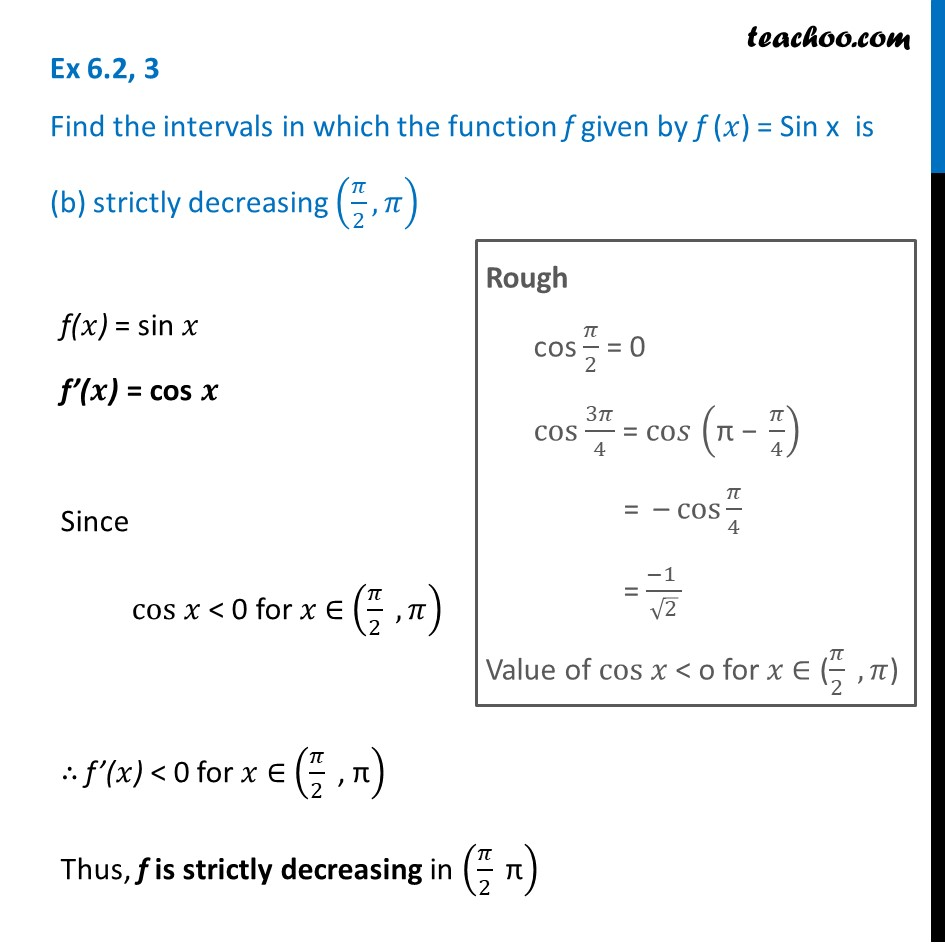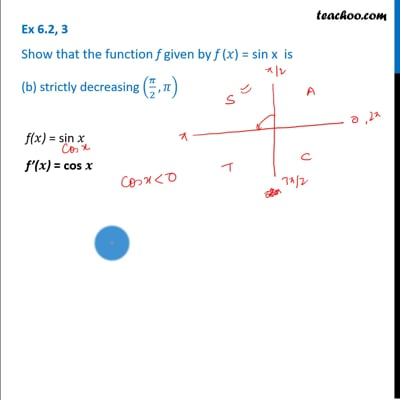This video is only available for Teachoo black users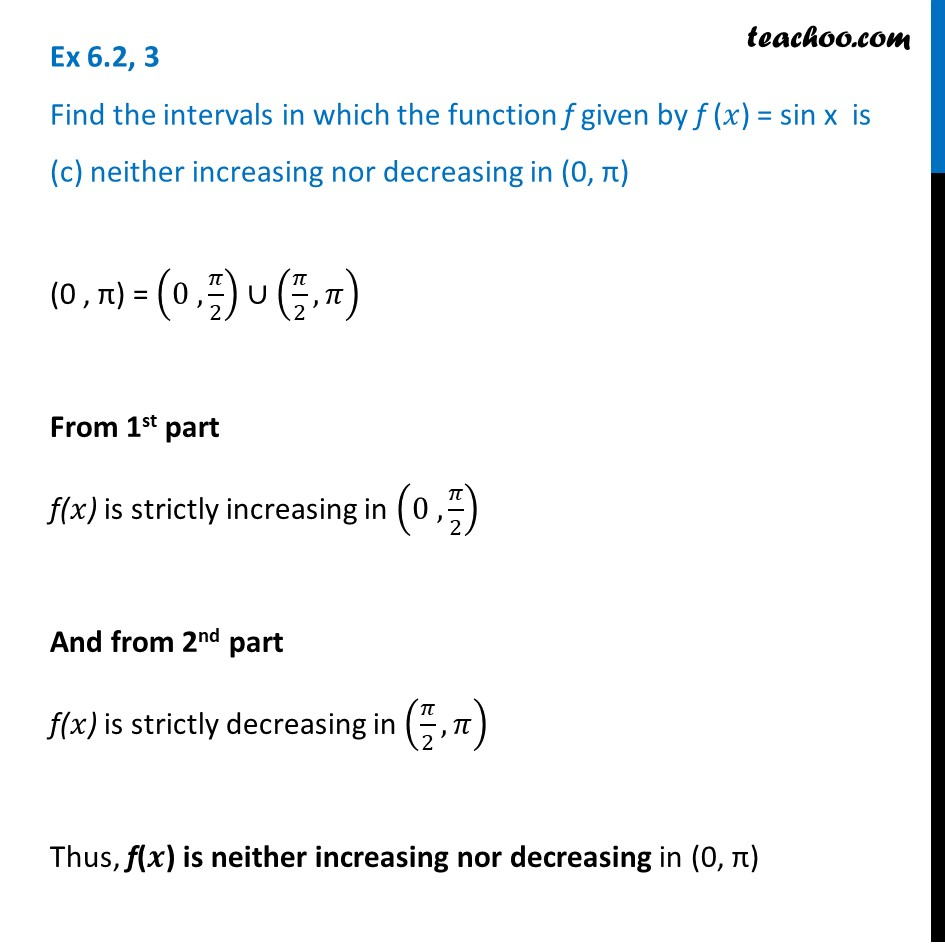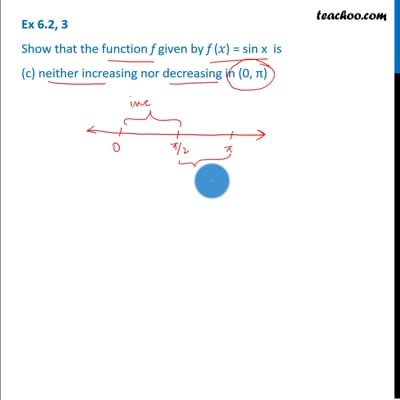This video is only available for Teachoo black users

Solve all your doubts with Teachoo Black (new monthly pack available now!)

### Transcript

Ex 6.2, 3 Find the intervals in which the function f given by f (𝑥) = sin 𝑥 is (a) strictly increasing in (0 , 𝜋/2) f(𝑥) = sin 𝑥 f’(𝒙) = cos 𝒙 Since cos 𝑥 > 0 for 𝑥 ∈ ("0 , " 𝜋/2) ∴ f’(𝑥) < 0 for 𝑥 ∈ (0 , π) Thus, f is strictly increasing in ("0 , " 𝜋/2) Rough cos 0 = 1 cos 𝜋/4 = 1/√2 cos 𝜋/2 = 0 Value of cos⁡𝑥 > 0 for (0 , 𝜋/2) Ex 6.2, 3 Find the intervals in which the function f given by f (𝑥) = Sin x is (b) strictly decreasing (𝜋/2,𝜋)f(𝑥) = sin 𝑥 f’(𝒙) = cos 𝒙 Since cos 𝑥 < 0 for 𝑥 ∈ (𝜋/2 , 𝜋) ∴ f’(𝑥) < 0 for 𝑥 ∈ (𝜋/2 " , π" ) Thus, f is strictly decreasing in (𝜋/2 " π" ) Rough cos 𝜋/2 = 0 cos 3𝜋/4 = co𝑠 ("π − " 𝜋/4) = – cos⁡𝜋/4 = (−1 )/√2 Value of cos 𝑥 < o for 𝑥 ∈ (𝜋/2 , 𝜋) Ex 6.2, 3 Find the intervals in which the function f given by f (𝑥) = sin x is (c) neither increasing nor decreasing in (0, π)(0 , π) = (0 , 𝜋/2) ∪ (𝜋/2,𝜋) From 1st part f(𝑥) is strictly increasing in (0 , 𝜋/2) And from 2nd part f(𝑥) is strictly decreasing in (𝜋/2,𝜋) Thus, f(𝒙) is neither increasing nor decreasing in (0, π)